Home Services Publications Contact usLanguages:English

 Link to: SONNE Technologies (Shanghai) Co., Ltd. ChenYang Technologies GmbH & Co. KG ChenYang Technologies Worldwide

 Legal Notice Disclaimer Delivery & Payment Data Protection General terms and conditions

 Employment Offer Feedback News of ChenYang Technologies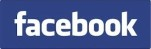Precise Parameter Determination of Damped Oscillation Signals

 Parameter determination of damped oscillation signals plays an important role in the technical diagnosis of analog circuits and systems, and for the measurement and analysis of elektrical and mechanical quantities. A typical damped sinusoid oscillation signal can usually be written by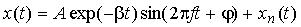(1) with A and j as beginning amplitude and phase, b as damping factor, and xn(t) as noise. The signal frequency f of the damped oscillation signal can firstly be estimated using the sampling data via more than 2 signal periods. The parameters b, A and j can then be determined by the following algorithm. For parameter estimation, the oscillation signal is sampled via 2 periods. Each period contains N samples. The sampling data are two-dimensionally numbered, i.e., x(i,j), i=0, 1, 2,..., N-1 and j=1, 2. The amplitudes A(k) and phases j(k), k=1,2, of each period are calculated by a discrete Fourier transform. The value of the beginning phase j * is resulted from the mean value: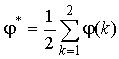(2)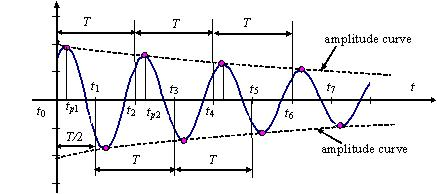Fig. 1 Estimation of b* and A* using the sampling data of the first two periods From Fig. 1 the damping factor b* can be derived as: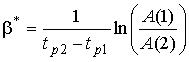(3) where tp1 and tp2 denote the time at the maximum of the sampled signal in the first and second period, respectively. The beginning amplitude A* is then determined by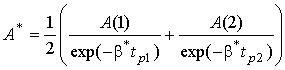(4) For practical uses the parameter estimation algorithm mentioned above is not satisfying with respect to its accuracy. The errors are caused by noise and the DFT due to signal damping and asynchronous sampling. The noise influence on the parameter estimation can be reduced by an averaging with the use of sampling data via more than 2 signal periods. The systematic errors should be reduced by the self-correction algorithm (Fig. 2). After a self-correction the parameters b, A, and j are determined according to the self-correction: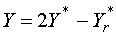(Y=b, A, and j)                      (5)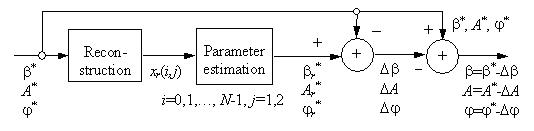Fig. 2 Self-correction algorithm for compensating systematic errors of parameter estimation The final output parameters of an iterative self-correction algorithm are written by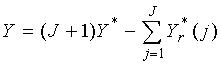(Y=b, A, and j) (6) with J as the number of iterative self-corrections and Y*r (j) as reference output parameters in the j-th self-correction. Different simulations are made by using the damped oscillation signal (1). The signal is sampled asynchronously. Fig. 3 shows the simulation results calculated by the parameter estimation algorithm without self-correction in the case of xn(t)=0. Calculation errors are caused by the original algorithm and the asynchronous sampling. They increase with the asynchronous factor a and decrease with the number of samples N per signal period. The errors mentioned above can be compensated by the self-correction algorithm. Fig. 4 shows the reduction errors of the parameters after the second iterative self-correction using (6) with J=2. The relative errors are limited within ±0.02% for determining all three parameters both using synchronous and asynchronous sampling data if noise is not considered.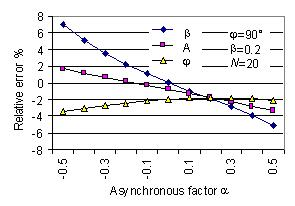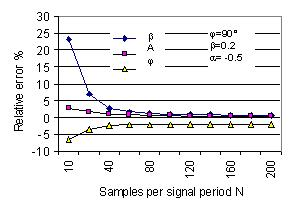Fig. 3  Parameter estimation errors of damped signal (1) calculated by algorithm without self-correction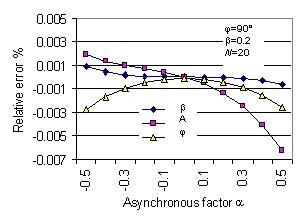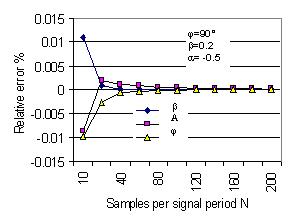Fig. 4  Parameter estimation errors of damped signal (1) calculated by algorithm with the self-correction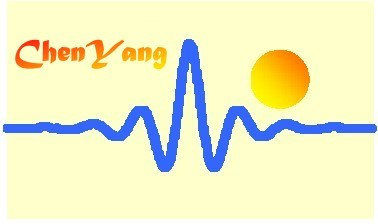©ChenYang Technologies GmbH & Co. KGMarkt Schwabener Str. 885464 Finsing, Germany Tel.: +49-(0)8121-2574100Fax: +49-(0)8121-2574101Email: info@chenyang.de